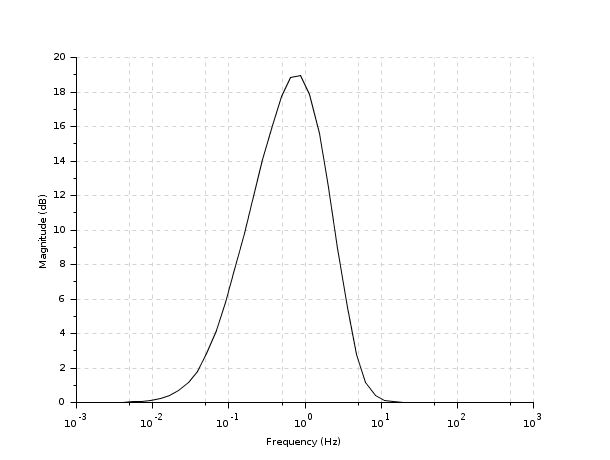Change language to:
Français - 日本語 - Português - Русский

See the recommended documentation of this function

# lqg

LQG compensator

### Calling Sequence

`[K]=lqg(P,r)`

### Arguments

P

`syslin` list (augmented plant) in state-space form

r

1x2 row vector = (number of measurements, number of inputs) (dimension of the 2,2 part of `P`)

K

`syslin` list (controller)

### Description

`lqg` computes the linear optimal LQG (H2) controller for the "augmented" plant `P=syslin('c',A,B,C,D)` (continuous time) or `P=syslin('d',A,B,C,D)` (discrete time).

The function `lqg2stan` returns `P` and `r` given the nominal plant, weighting terms and variances of noises.

`K` is given by the following ABCD matrices: `[A+B*Kc+Kf*C+Kf*D*Kc,-Kf,Kc,0]` where `Kc=lqr(P12)` is the controller gain and `Kf=lqe(P21)` is the filter gain. See example in `lqg2stan`.

### Examples

```s=poly(0,'s');
Plant=syslin('c',[1/(s+1)*s/(s-1)^2]);     //Nominal Plant
P22=tf2ss(Plant);    //...in state-space form
[ny,nu,nx]=size(P22);
rand('seed',0);rand('normal');
bigQ=rand(nx+nu,nx+nu);
bigQ=bigQ*bigQ';
bigR=rand(nx+ny,nx+ny);
bigR=bigR*bigR';  //random weighting matrices
[Plqg,r]=lqg2stan(P22,bigQ,bigR);     //LQG pb as a standard problem
Klqg=lqg(Plqg,r);          //Controller
spec(h_cl(Plqg,r,Klqg))    //Check internal stability
[Slqg,Rlqg,Tlqg]=sensi(P22,Klqg);  //Sensitivity functions
frq=logspace(-3,3);     //10^-3  to 10^3
y=svplot(Slqg);       //Computes singular values;
gainplot(frq,y)   //Plot sing. values```